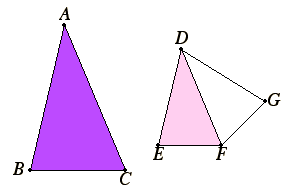# Proposition 6

If two triangles have one angle equal to one angle and the sides about the equal angles proportional, then the triangles are equiangular and have those angles equal opposite the corresponding sides.

Let ABC and DEF be two triangles having one angle BAC equal to one angle EDF and the sides about the equal angles proportional, so that BA is to AC as ED is to DF.

I say that the triangle ABC is equiangular with the triangle DEF, and has the angle ABC equal to the angle DEF, and the angle ACB equal to the angle DFE.I.23

On the straight line DF and at the points D and F on it, construct the angle FDG equal to either of the angles BAC or EDF, and the angle DFG equal to the angle ACB.

I.32

Therefore the remaining angle at B equals the remaining angle at G. Therefore the triangle ABC is equiangular with the triangle DGF.

VI.4

Therefore, proportionally BA is to AC as GD is to DF.

V.11

But, by hypothesis, BA is to AC also as ED is to DF, therefore also ED is to DF as GD is to DF.

Therefore ED equals GD. And DF is common, therefore the two sides ED and DF equal the two sides GD and DF, and the angle EDF equals the angle GDF, therefore the base EF equals the base GF, the triangle DEF equals the triangle DGF, and the remaining angles equal the remaining angles, namely those opposite the equal sides.

Therefore the angle DFG equals the angle DFE, and the angle DGF equals the angle DEF.

But the angle DFG equals the angle ACB, therefore the angle ACB also equals the angle DFE.

I.32

And, by hypothesis, the angle BAC also equals the angle EDF, therefore the remaining angle at B also equals the remaining angle at E. Therefore the triangle ABC is equiangular with the triangle DEF.

Therefore, if two triangles have one angle equal to one angle and the sides about the equal angles proportional, then the triangles are equiangular and have those angles equal opposite the corresponding sides.

Q.E.D.

## Guide

This is a side-angle-side similarity theorem analogous to side-angle-side congruence theorem I.4.

Here’s a summary of the proof. Construct a triangle DGF equiangular with triangle ABC. Then triangle DGF is similar to triangle ABC ( VI.4), and that gives us the proportion

BA : AC = GD : DF.

But we have assumed the proportion

BA : AC = ED : DF,

and these two proportions together give us

GD : DF = ED : DF

(V.11), from which it follows that GD = ED (V.9). Therefore triangles DEF and DGF are congruent, and the rest follows easily.

#### Use of this proposition

This proposition is used in the proofs of propositions VI.20, VI.32, XII.1, and several times in XII.12.